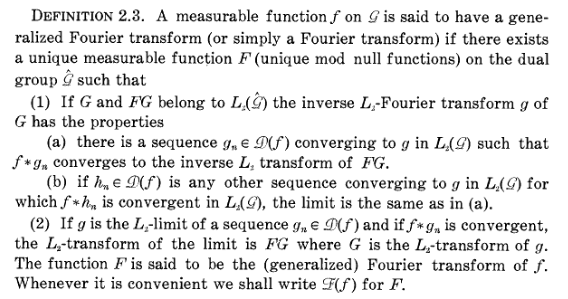# Characterization of the Laplace Transform

One of the main properties of the Laplace transform is given by the convolution theorem. $$\mathcal{L}(f*g)=\mathcal{L}(f)\cdot\mathcal{L}(g)$$ Question: Is there a full characterization of the Laplace transform based on this property? I have in mind a theorem that reads: "Let $\mathcal{N}$ be an operator with the convolution property (given above). If $\mathcal{N}$ also satisfies properties A,B,C then $\mathcal{N}=\mathcal{L}$."

This operational approach to a definition of Fourier/Laplace transforms has been developed by R.A. Kunze, An operator theoretic approach to generalized Fourier transforms.

This is an attempt to give an intrinsic definition generalizing the conditions under which a pair of functions on the line may be said to be Fourier transforms of each other, in a way which is independent of any special methods of summation and more inclusive than the usual $$L_p$$ theory. Our approach builds on earlier work by I.E. Segal, who suggested the definition "a measurable function $$f$$ has a generalized Fourier transform if the operation of convolution by $$f$$ in $$L_2$$ has a normal extension."

Kunze's definition of the generalized Fourier transform is contained in the following:For an extension of this approach from the real line to compact Abelian groups, see K.I. Gross, Generalized Fourier Transforms of Distributions.

• Thanks for the reference. I can see that it is related, but I don't immediately see if it answers the question. He seems to define the Fourier transform in terms of preserving convolution. Does that mean that this is the only relevant property for the Fourier transform, besides linearity? Is it immediate that this also applies to the Laplace Transform? Oct 11 '13 at 18:26
• Corollary 3.3.4 of Kunze actually derives the linearity of the Fourier transform from its definition as a mapping that transforms convolution into multiplication, so I would think that there is no need to invoke the linearity as an additional requirement. Oct 11 '13 at 19:17
• I see. Maybe I can phrase my confusion as follows: It seems possible that the convolution property above is, after all, all you need to characterize the Fourier transform. I cannot see that the paper answers the following two questions: 1-Does this property uniquely define the Fourier transform? 2-What happens when we restrict the set over which we define the operator, for example, if we only consider real-valued functions. Oct 12 '13 at 18:07